# Proof of Circle Theorems

## Arrange the stages of the proofs for the standard circle theorems in the correct order.

##### Circle TheoremsHelp VideoMore on CirclesMore on Angles

Drag the statements proving the theorem into the correct order.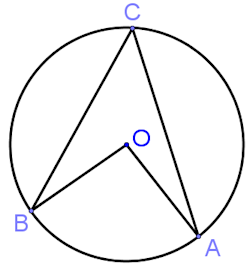Similarly ∠AOC = 180° – 2 x ∠OCAOB = OC (radii of circle)∠BOA = 2∠BCA Q.E.D.Construct radius OC∠COB = 180° – 2 x ∠BCO (Angle sum of triangle OBC)To prove: ∠BOA = 2∠BCA∠BCO = ∠OBC (equal angles in isosceles triangle)∠BOA = 2(∠BCO + ∠OCA)∠BOA = 360° – (180° – 2 x ∠BCO + 180° – 2 x ∠OCA)∴ OBC is an isosceles triangle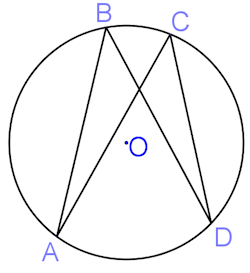∴ 2 x ∠ABD = 2 x ∠ACD∠AOD = 2 x ∠ABD (angle at centre twice angle at circumference)∠AOD = 2 x ∠ACD (angle at centre twice angle at circumference)∠ABD = ∠ACD Q.E.D.Construct radii from A and DTo prove: ∠ABD = ∠ACD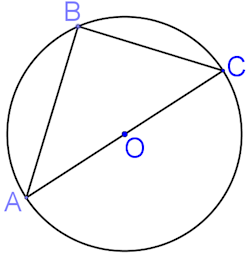Similarly in triangle BCO ∠OCB = ∠OBCTo prove: ∠ABC = 90°∴ ∠OAB = ∠OBA (equal angles in isosceles triangle ABO)∠OAB + ∠OBA + ∠OCB + ∠OBC = 180° (Angle sum of triangle ABC)OA = OB (radii)∴ 2(∠OBA + ∠OBC) = 180°∴ ABO is an isosceles triangle (two equal sides)∠ABC = 90° Q.E.D.Construct the radius OB∴ ∠OBA + ∠OBC = 90°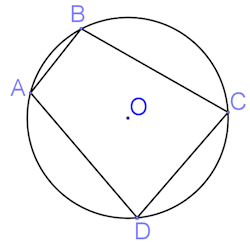The obtuse and reflex angles at O add up to 360° (angles at a point)Similarly the obtuse angle AOC = 2 x ∠CDATo prove ∠ABC + ∠CDA = 180°∴ 2 x ∠ABC + 2 x ∠CDA = 360°Reflex ∠AOC = 2 x ∠ABC (angle at centre twice angle at circumference)∠ABC + ∠CDA = 180° Q.E.D.Construct the radii OA and OC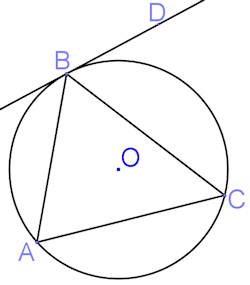2 x ∠CAB = 2 x ∠CBD (from  above)∠OBC + ∠CBD = 90° (angle between radius and tangent) ∠CAB = ∠CBD Q.E.D.2 x ∠OBC + ∠COB = 180° (angle sum of triangle) Obtuse ∠COB = 2 x ∠CAB (angle at centre twice angle at circumference) ∠COB = 2 x ∠CBDTo prove ∠CAB = ∠CBD∠OBC = ∠OCB (equal angles in isosceles triangle OBC)Construct the radii OB and OC2 x ∠OBC + ∠COB = 2(∠OBC + ∠CBD) (from  and  above)Check

This is Proof of Circle Theorems level 0. You can also try:

## Instructions

Try your best to answer the questions above. Type your answers into the boxes provided leaving no spaces. As you work through the exercise regularly click the "check" button. If you have any wrong answers, do your best to do corrections but if there is anything you don't understand, please ask your teacher for help.

When you have got all of the questions correct you may want to print out this page and paste it into your exercise book. If you keep your work in an ePortfolio you could take a screen shot of your answers and paste that into your Maths file.

## Transum.org

This web site contains over a thousand free mathematical activities for teachers and pupils. Click here to go to the main page which links to all of the resources available.## More Activities:

Mathematicians are not the people who find Maths easy; they are the people who enjoy how mystifying, puzzling and hard it is. Are you a mathematician?

Comment recorded on the 14 October 'Starter of the Day' page by Inger Kisby, Herts and Essex High School:

"Just a quick note to say that we use a lot of your starters. It is lovely to have so many different ideas to start a lesson with. Thank you very much and keep up the good work."

Comment recorded on the 17 November 'Starter of the Day' page by Amy Thay, Coventry:

"Thank you so much for your wonderful site. I have so much material to use in class and inspire me to try something a little different more often. I am going to show my maths department your website and encourage them to use it too. How lovely that you have compiled such a great resource to help teachers and pupils.
Thanks again"

#### Hi-Low Predict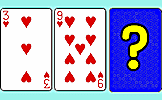A version of the Play Your Cards Right TV programme. Calculate the probabilities of cards being higher or lower than the one shown. a fun way to practise applying probability and using fractions.

There are answers to this exercise but they are available in this space to teachers, tutors and parents who have logged in to their Transum subscription on this computer.

A Transum subscription unlocks the answers to the online exercises, quizzes and puzzles. It also provides the teacher with access to quality external links on each of the Transum Topic pages and the facility to add to the collection themselves.

Subscribers can manage class lists, lesson plans and assessment data in the Class Admin application and have access to reports of the Transum Trophies earned by class members.

Subscribe

## Go Maths

Learning and understanding Mathematics, at every level, requires learner engagement. Mathematics is not a spectator sport. Sometimes traditional teaching fails to actively involve students. One way to address the problem is through the use of interactive activities and this web site provides many of those. The Go Maths page is an alphabetical list of free activities designed for students in Secondary/High school.

## Maths Map

Are you looking for something specific? An exercise to supplement the topic you are studying at school at the moment perhaps. Navigate using our Maths Map to find exercises, puzzles and Maths lesson starters grouped by topic.

## Teachers

If you found this activity useful don't forget to record it in your scheme of work or learning management system. The short URL, ready to be copied and pasted, is as follows:

Do you have any comments? It is always useful to receive feedback and helps make this free resource even more useful for those learning Mathematics anywhere in the world. Click here to enter your comments.For All: